Related Articles
Class 8 NCERT Solutions – Chapter 11 Mensuration – Exercise 11.2
• Last Updated : 24 Nov, 2020

### Question 1. The shape of the top surface of a table is a trapezium. Find its area if its parallel sides are 1 m and 1.2 m and the perpendicular distance between them is 0.8 m.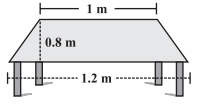Solution:

One parallel side of the trapezium (a) = 1 m

And second side (b) = 1.2 m and

height (h) = 0.8 m

Area of top surface of the table = (½) × (a + b)h

= (½) × (1 + 1.2)0.8

=  (½) × 2.2 × 0.8

= 0.88

Area of top surface of the table is 0.88 m2 .

### Question 2. The area of a trapezium is 34  cm2 and the length of one of the parallel sides is 10 cm and its height is 4 cm Find the length of the other parallel side.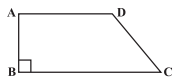Solution:

Let the length of the other parallel side be b.

Length of one parallel side, a = 10 cm

height, (h) = 4 cm and

Area of a trapezium is 34 cm2

Formula for, Area of trapezium = (1/2) × (a + b)h

34 = ½(10 + b) × 4

34 = 2 × (10 + b)

After simplifying, b = 7

Hence another required parallel side is 7 cm.

### Question 3. The length of the fence of a trapezium-shaped field ABCD is 120 m. If BC = 48 m, CD = 17 m and AD = 40 m, find the area of this field. Side AB is perpendicular to the parallel sides AD and BC.

Solution:

Given: BC = 48 m, CD = 17 m,

AD = 40 m and perimeter = 120 m

∵ Perimeter of trapezium ABCD

= AB + BC + CD + DA

120 = AB + 48 + 17 + 40

120 = AB = 105

AB = 120 – 105 = 15 m

Now, Area of the field = (½) × (BC + AD) × AB

= (½) × (48 + 40) × 15

= (½) × 88 × 15

= 660

Hence, area of the field ABCD is 660m2

### Question 4. The diagonal of a quadrilateral shaped field is 24 m and the perpendiculars dropped on it from the remaining opposite vertices are 8 m and 13 m. Find the area of the field.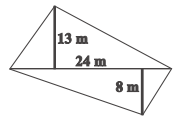Solution:

Consider, h1 = 13 m, h2 = 8 m and AC = 24 m

Area of quadrilateral ABCD = Area of triangle ABC + Area of triangle ADC

= ½(bh1) + ½(bh2)

= ½ × b(h1 + h2) = (½) × 24 × (13 + 8)

= (½) × 24 × 21 = 252

Hence, required area of the field is 252 m2

### Question 5. The diagonals of a rhombus are 7.5 cm and 12 cm. Find its area.

Solution:

Given:  d1 = 7.5 cm and d2 = 12 cm

We know that,Area of rhombus =  (½) × d1 × d2 = (½) × 7.5 × 12 = 45

Therefore, area of rhombus is 45 cm2 .

### Question 6. Find the area of a rhombus whose side is 5 cm and whose altitude is 4.8 cm. If one of the diagonals is 8 cm long, find the length of the other diagonal.

Solution:

Since a rhombus is also a kind of a parallelogram.

Formula for Area of rhombus = Base × Altitude

Putting values, we have

Area of rhombus = 6 × 4 = 24

Area of rhombus is 24 cm2

Also, Formula for Area of rhombus = (½) × d1d2

After substituting the values, we get

24 = (½) × 8 × d2

d2 = 6

Hence, the length of the other diagonal is 6 cm.

### Question 7. The floor of a building consists of 3000 tiles which are rhombus-shaped and each of its diagonals is 45 cm and 30 cm in length. Find the total cost of polishing the floor, if the cost per m2 is Rs. 4.

Solution:

Length of one diagonal,  d1 = 45 cm and  d2= 30 cm

∵ Area of one tile =  (½)d1d2 = (½) × 45 × 30 = 675

Area of one tile is 675 cm2

Area of 3000 tiles is

= 675 × 3000

= 2025000 cm2

= 2025000/10000

= 202.50 m2 [∵ 1m2 = 10000 cm2]

∵ Cost of polishing the floor per sq. meter = 4

Cost of polishing the floor per 202.50 sq. meter = 4 × 202.50 = 810

Hence the total cost of polishing the floor is Rs. 810.

### Question 8. Mohan wants to buy a trapezium-shaped field. Its side along the river is parallel to and twice the side along the road. If the area of this field is 10500 m2 and the perpendicular distance between the two parallel sides is 100 m, find the length of the side along the river.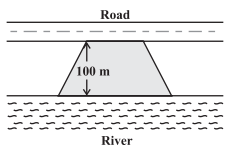Solution:

Perpendicular distance  (h) = 100 m (Given)

Area of the trapezium shaped field = 10500 m2 (Given)

Let side along the road be ‘x’ m and side along the river =  2x m

Area of the trapezium field =  (½) × (a + b) × h

10500 =  (½) × (x + 2x) × 100

10500 = 3x × 50

After simplifying, we have x = 70, which means side along the river is 70 m

Hence, the side along the river =  2x = 2(70) = 140 m.

### Question 9. The top surface of a raised platform is in the shape of a regular octagon as shown in the figure. Find the area of the octagonal surface.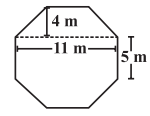Solution: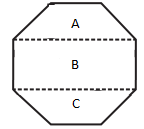Octagon having eight equal sides, each 5 m. (given)

Divide the octagon as show in the below figure, 2 trapeziums whose parallel and perpendicular sides are 11 m and 4 m respectively and 3rd one is rectangle having length and breadth 11 m and 5 m respectively.

Now, Area of two trapeziums = 2 [(½) × (a + b) × h]

= 2 × (½) × (11 + 5 ) × 4

= 4 × 16 = 64

Area of two trapeziums is 64 m2

Also, Area of rectangle = length × breadth

= 11 × 5 = 55

Area of rectangle is 55 m2

Total area of octagon = 64 + 55

= 119 m2

### Question 10. There is a pentagonal shaped park as shown in the figure.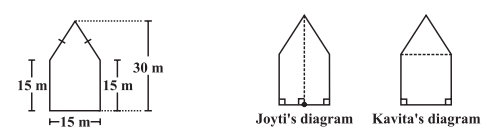### For finding its area Jyoti and Kavita divided it in two different ways. Find the area of this park using both ways. Can you suggest some other way of finding its area?

Solution:

First way:  By Jyoti’s diagram,

Area of pentagon = Area of trapezium ABCP + Area of trapezium AEDP

=  (½)(AP + BC) × CP + (1/2) × (ED + AP) × DP

=  (½)(30 + 15) × CP + (1/2) × (15 + 30) × DP

=  (½) × (30 + 15) × (CP + DP)

=  (½) × 45 × CD

= (1/2) × 45 × 15

= 337.5 m2

Area of pentagon is 337.5 m2

Second way: By Kavita’s diagram

Here, a perpendicular AM drawn to BE.

AM = 30 – 15 = 15 m

Area of pentagon = Area of  triangle ABE + Area of square BCDE (from above figure)

= (½) × 15 × 15 + (15 × 15)

= 112.5 + 225.0

= 337.5

Hence, total area of pentagon shaped park = 337.5 m2

### Question 11. The Diagram of the adjacent picture frame has outer dimensions = 24 cm × 28 cm and inner dimensions 16 cm × 20 cm. Find the area of each section of the frame, if the width of each section is the same.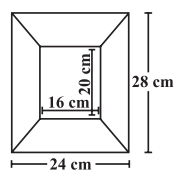Solution: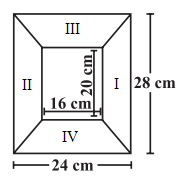Divide given figure into 4 parts, as shown below:

Here two of given figures (I) and (II) are similar in dimensions.

And also figures (III) and (IV) are similar in dimensions.

Area of figure (I) = Area of trapezium

=  (½) × (a + b) × h

= (½) × (28 + 20) × 4

=  (½) × 48 × 4 = 96

Area of figure (I) = 96 cm2

Also, Area of figure (II) = 96 cm2

Now, Area of figure (III) = Area of trapezium

= (½) × (a + b) × h

= (½) × (24 + 16)4

= (½) × 40 × 4 = 80

Area of figure (III) is 80 cm2

Also, Area of figure (IV) = 80 cm2

My Personal Notes arrow_drop_up